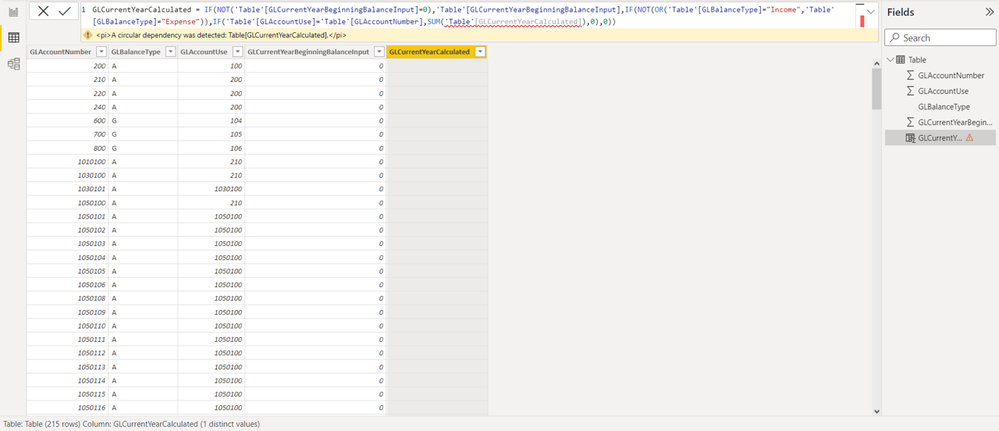cancel
Showing results for
Did you mean:

Fabric is Generally Available. Browse Fabric Presentations. Work towards your Fabric certification with the Cloud Skills Challenge.Anonymous
Not applicable

## Circular dependency error... from data in a same column?

Hey, community! I have some trouble with a "Circular dependency", let me tell you about it:

In Microsoft Excel I am considering columns and cells to make specific sums in a calculated column, here's an image as an example where the column "G" is where I want to show the result of the sums made:Now, in Microsoft Excel there's no trouble with it, everything is fine, the real problem is when I want to do the same thing in Power BI because the app tells me there's an error called "Circular dependency". Here's the image that shows what's going on:So, my two questions are:

1. How can I avoid "Circular dependency" in Power BI so the values in the same column where I am making sums can also add themselves?
2. Taking as an example the image in Microsoft Excel, if the number from the cell "C2" can be found several times in column "E", the respective amounts from the column "G" will sum, is there a way in Power BI where I can select a whole column and a single cell from a column at the same time? (from what I've tried, I can only select columns, but I want to select cells or rows as well)

I'm expecting that the calculated column from my Power BI tabular model show the sums corresponding to the conditions I'm asking: "If the IDs from a column match the ID from a single row, the respective amounts that match will sum, ending this cycle by not finding the next ID and showing me as a result the final amount from that particular row".

Thank you.

P.S.: Here's the formula made in Excel:

`=IF(NOT(F2=0),F2,IF(NOT(OR(D2="I",D2="G")),SUMIF(\$E\$2:\$E\$1048576,C2,\$G\$2:\$G\$1048576),0))`

And here's the formula made in Power BI:

`GLCurrentYearCalculated = IF(NOT('Table'[GLCurrentYearBeginningBalanceInput]=0),'Table'[GLCurrentYearBeginningBalanceInput],IF(NOT(OR('Table'[GLBalanceType]="Income",'Table'[GLBalanceType]="Expense")),IF('Table'[GLAccountUse]='Table'[GLAccountNumber],SUM('Table'[GLCurrentYearCalculated]),0),0))`
2 REPLIES 2Super User

@Anonymous , You can not same column in power bi like excel.

Also, you can not use the last row of the column you are building.

you can use it earlier to work across rows. You can use a cumulative formula  to get data

Can you share sample data and sample output in table format? Or a sample pbix after removing sensitive data.Anonymous
Not applicable

Sure! Here's the Power BI document where the data and the calculated column are included.

An observation of the data is the following:

- In the data we have "AccountNumber" and "AccountNumberAffected", the first one is the ID of an account and the second one is the corresponding ID of the account that affects. For example: If I have operations with the account "240" then I know that it will affect the result from the account "200".

Knowing this, here's the next thing:

- The user can enter a "BeginningBalance" for a specific account, if we don't have a user input then by default the beggining balance is "0".

And finally, what I want to do is this:

- Knowing that we have user inputs and "0" values in the "BeginningBalanceUserInput" field, in the calculated column I want to do two things: IF the "BeginningBalanceUserInput" is not "0" then just put the same value given, but if it's "0" then SUM the "BeginningBalanceUserInput" values knowing that one account affects the other depending on their corresponding IDs.

Thank you 😄Announcements#### Power BI Monthly Update - November 2023

Check out the November 2023 Power BI update to learn about new features.#### Fabric Community News unified experience

Read the latest Fabric Community announcements, including updates on Power BI, Synapse, Data Factory and Data Activator.#### The largest Power BI and Fabric virtual conference

130+ sessions, 130+ speakers, Product managers, MVPs, and experts. All about Power BI and Fabric. Attend online or watch the recordings.Top Solution Authors
Top Kudoed Authors
Users online (1,836)